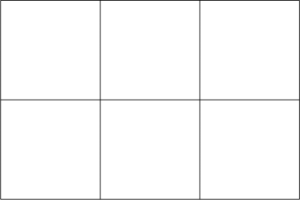시간 제한메모리 제한제출정답맞힌 사람정답 비율
2 초 512 MB46323274.419%

## 문제We have found: 6 rectangles 1 × 1, 4 rectangles 2 × 1, 3 rectangles 1 × 2, 2 rectangles 2 × 2, 2 rectangles 3 × 1 and one rectangle 3 × 2, what gives 18 in total. Clearly, we are interested in rectangles with vertices in grid points, i.e., the points located at the intersections of vertical and horizontal line segments, and having vertical or horizontal sides. The above grid has dimensions 3 × 2.

And how many such rectangles of perimeter at least 6 can be found in the figure? You can find the answer in the Example section.

## 입력

The first and only line of the standard input contains three integers: n, m and p (1 ≤ n, m ≤ 5,000, 4 ≤ p ≤ 2(n+m)), representing the dimensions of the grid and the lower bound for the perimeter of the rectangles.

## 출력

In the first line of the standard output your program should output one integer: the number of rectangles with vertices in grid points of the grid n × m, having vertical or horizontal sides and which perimeter is at least p.

## 예제 입력 1

3 2 4


## 예제 출력 1

18


## 예제 입력 2

3 2 6


## 예제 출력 2

12